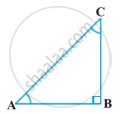Share

Concept: Trigonometric Ratios of Complementary Angles

description

We know that complementary angles are the set of two angles such that their sum is equal to 90°. For example: 30° and 60° are complementary to each other as their sum is equal to 90°.The triangle ∆ABC given below, is right angled at B; ∠A and ∠C form a complementary pair.

notes

Recall that two angles are said to be complementary if their sum equals 90°. In

ABC, right-angled at B.Let ∠A= θ

In ∆ABC,

A+∠B+∠C= 180° (Angle sum property)

θ+ 90°+∠C= 180°

C= 180°-90°-θ

C= (90°-θ)

Trigonometric ratios-

1) sin(90°-θ)= "AB"/"AC"= cosθ

2) cos(90°-θ)= "BC"/"AC"= sinθ

3)tan(90°-θ)= "AB"/"BC"= cotθ

4)cot(90°-θ)= "BC"/"AB"= tanθ

5)sec(90°-θ)= "AC"/"BC"= cosecθ

6)cosec(90°-θ)= "AC"/"AB"= secθ

Example: Evaluate "sin18°"/"cos72°"

solutioin- "sin(90°-72°)"/"cos72°"

sin(90°-θ)= cosθ= "cos72°"/"cos72°"= 1

Video Tutorials

We have provided more than 1 series of video tutorials for some topics to help you get a better understanding of the topic.

Series 1

Series 2

Shaalaa.com

Trigonometric Identities part 1 (Ratio complementary angle) [00:10:14]
S
1
0%

S